Maths-
General
Easy

Question

# The sum of the digits of a two-digit number is 7. If the digits are reversed, the number is reduced by 27. Find the number?Hint:

## The correct answer is: 2 sisters

### Ans :- 52 is the number which satisfies the given conditions.Explanation :-let the number be xy i.e 10x + y = xy where x and y are digits of the numberStep 1:- Find the equation using sum of digits = 7Sum of digits = 7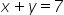— Eq1Step 2:- Find the equation using the 2nd condition.If the digits are reversed, the number is reduced by 27.xy - yx = 27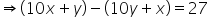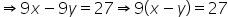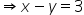— Eq2Step 3:- Find x by eliminating yAdding Eq1 and Eq2We get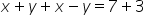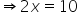∴x = 5 Step 4:- Find the value of y by substituting the x =5 in Eq1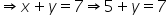∴y = 2 ∴ number xy = 52 is the number that satisfies the given condition.#### With Turito Foundation.#### Get an Expert Advice From Turito.